# 简介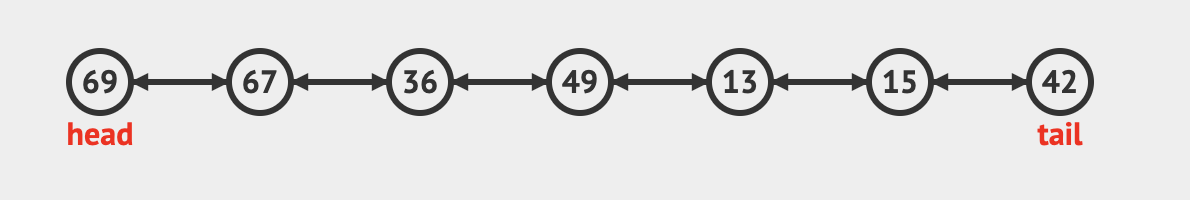``````public class DoublyLinkedList {

class Node {
int data;
Node next;
Node prev;
//Node的构造函数
Node(int d) {
data = d;
}
}
}
``````

## 头部插入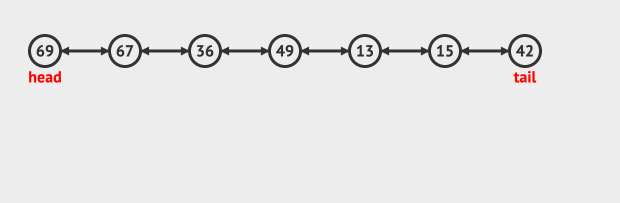``````    //插入到linkedList的头部
public void push(int newData) {
//构建要插入的节点
Node newNode = new Node(newData);
//新节点的prev指向null
newNode.prev = null;

}
``````

## 尾部插入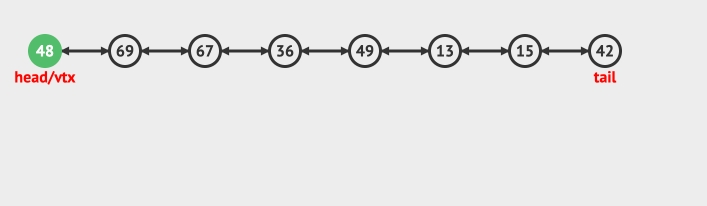``````   //新节点插入到list最后面
public void append(int newData) {
//创建新节点
Node newNode = new Node(newData);
newNode.prev = null;
return;
}

newNode.next = null;
//找到最后一个节点
while (last.next != null) {
last = last.next;
}
//插入
last.next = newNode;
newNode.prev = last;
return;
}
``````

## 插入给定的位置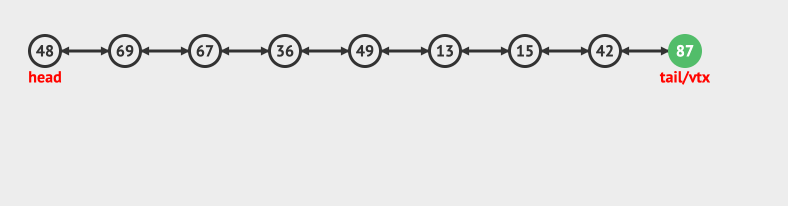``````    //插入在第几个元素之后
public void insertAfter(int index, int newData) {
for (int i = 1; i < index; i++) {
if (prevNode == null) {
System.out.println("输入的index有误，请重新输入");
return;
}
prevNode = prevNode.next;
}
//创建新的节点
Node newNode = new Node(newData);
//新节点的next指向prevNode的下一个节点
newNode.next = prevNode.next;
//将新节点插入在prevNode之后
prevNode.next = newNode;
//将新节点的prev指向prevNode
newNode.prev = prevNode;

//newNode的下一个节点的prev指向newNode
if (newNode.next != null)
newNode.next.prev = newNode;
}
``````

## 删除指定位置的节点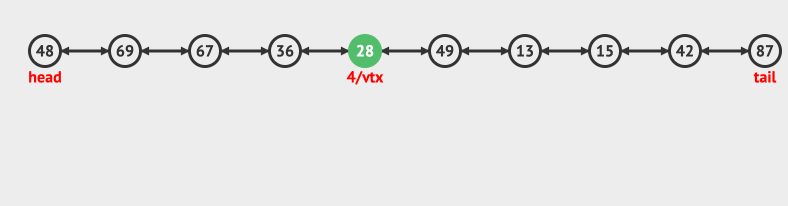``````    //删除特定位置的节点
void deleteNode(int index)
{
// 如果是空的，直接返回
return;

if (index == 1)
{
return;
}

// 找到要删除节点的前一个节点
for (int i=1; temp!=null && i<index-1; i++)
temp = temp.next;

// 如果超出范围
if (temp == null || temp.next == null)
return;

// temp->next 是要删除的节点，删除节点
Node next = temp.next.next;
temp.next = next;
Node prev = temp.next.prev;
prev.prev = prev;
}
``````

learn-algorithm